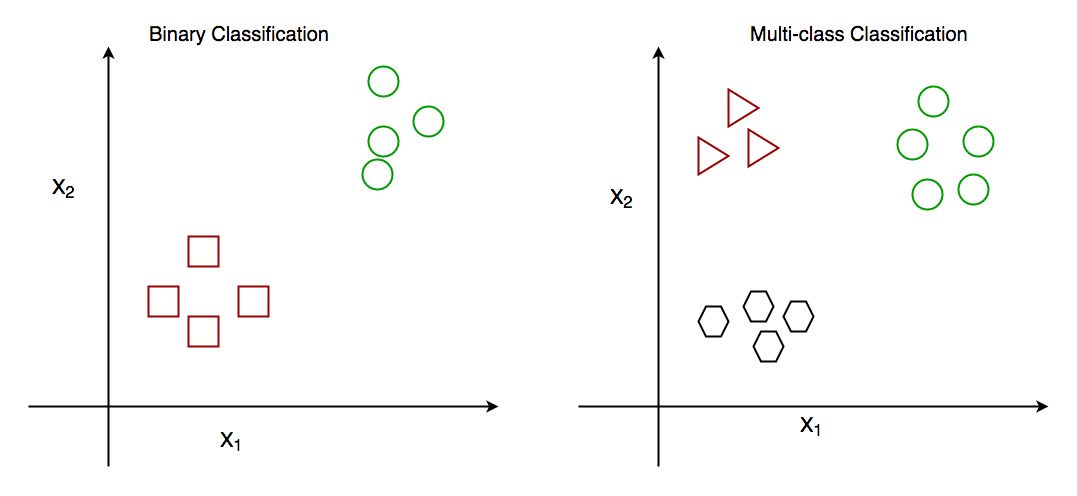GeeksforGeeks App
Open AppBrowser
Continue

# One-vs-Rest strategy for Multi-Class Classification

Prerequisite: Getting Started with Classification/

Classification is perhaps the most common Machine Learning task. Before we jump into what One-vs-Rest (OVR) classifiers are and how they work, you may follow the link below and get a brief overview of what classification is and how it is useful.

In general, there are two types of classification algorithms:

1. Binary classification algorithms.
2. Multi-class classification algorithms.

Binary classification is when we have to classify objects into two groups. Generally, these two groups consist of ‘True’ and ‘False’. For example, given a certain set of health attributes, a binary classification task may be to determine whether a person has diabetes or not.

On the other hand, in multi-class classification, there are more than two classes. For example, given a set of attributes of fruit, like it’s shape and colour, a multi-class classification task would be to determine the type of fruit.So, now that you have an idea of how binary and multi-class classification work, let us get on to how the one-vs-rest heuristic method is used.

One-vs-Rest (OVR) Method:
Many popular classification algorithms were designed natively for binary classification problems. These algorithms include :

• Logistic Regression
• Support Vector Machines (SVM)
• Perceptron Models

and many more.

So, these popular classification algorithms cannot directly be used for multi-class classification problems. Some heuristic methods are available that can split up multi-class classification problems into many different binary classification problems. To understand how this works, let us consider an example : Say, a classification problem is to classify various fruits into three types of fruits: banana, orange or apple. Now, this is clearly a multi-class classification problem. If you want to use a binary classification algorithm like, say SVM. The way One-vs-Rest method will deal with this is illustrated below :

Since there are three classes in the classification problem, the One-vs-Rest method will break down this problem into three binary classification problems:

• Problem 1 : Banana vs [Orange, Apple]
• Problem 2 : Orange vs [Banana, Apple]
• Problem 3 : Apple vs [Banana, Orange]

So instead of solving it as (Banana vs Orange vs Apple), it is solved using three binary classification problems as shown above.

A major downside or disadvantage of this method is that many models have to be created. For a multi-class problem with ‘n’ number of classes, ‘n’ number of models have to be created, which may slow down the entire process. However, it is very useful with datasets having a small number of classes, where we want to use a model like SVM or Logistic Regression.

Implementation of One-vs-Rest method using Python3
Python’s scikit-learn library offers a method OneVsRestClassifier(estimator, *, n_jobs=None) to implement this method. For this implementation, we will be using the popular ‘Wine dataset’, to determine the origin of wines using chemical attributes. We can direct this dataset using scikit-learn. To know more about this dataset, you can use the link below : Wine Dataset

We will use a Support Vector Machine, which is a binary classification algorithm and use it with the One-vs-Rest heuristic to perform multi-class classification.

To evaluate our model, we will see the accuracy score of the test set and the classification report of the model.

 `from` `sklearn.datasets ``import` `load_wine``from` `sklearn.multiclass ``import` `OneVsRestClassifier``from` `sklearn.svm ``import` `SVC``from` `sklearn.metrics ``import` `accuracy_score, classification_report``from` `sklearn.model_selection ``import` `train_test_split``import` `warnings``  ` `''' ``We are ignoring warnings because of a peculiar fact about this``dataset. The 3rd label, 'Label2' is never predicted and so the python ``interpreter throws a warning. However, this can safely be ignored because ``we are not concerned if a certain label is predicted or not ``'''``warnings.filterwarnings(``'ignore'``)``  ` `# Loading the dataset``dataset ``=` `load_wine()``X ``=` `dataset.data``y ``=` `dataset.target``  ` `# Splitting the dataset into training and testing sets``X_train, X_test, y_train, y_test ``=` `train_test_split(``    ``X, y, test_size ``=` `0.1``, random_state ``=` `13``)``  ` `# Creating the SVM model``model ``=` `OneVsRestClassifier(SVC())``  ` `# Fitting the model with training data``model.fit(X_train, y_train)``  ` `# Making a prediction on the test set``prediction ``=` `model.predict(X_test)``  ` `# Evaluating the model``print``(f"Test ``Set` `Accuracy : {accuracy_score(``    ``y_test, prediction) ``*` `100``} ``%``\n\n")``print``(f"Classification Report : \n\n{classification_report(``    ``y_test, prediction)}")`

Output:

```Test Set Accuracy : 66.66666666666666 %

Classification Report :

precision    recall  f1-score   support

0       0.62      1.00      0.77         5
1       0.70      0.88      0.78         8

micro avg       0.67      0.92      0.77        13
macro avg       0.66      0.94      0.77        13
weighted avg       0.67      0.92      0.77        13
```

We get a test set accuracy of approximately 66.667%. This is not bad for this dataset. This dataset is notorious for being difficult to classify and the benchmark accuracy is 62.4 +- 0.4 %. So, our result is actually quite good.

Conclusion:
Now that you know how to use the One-vs-Rest heuristic method for performing multi-class classification with binary classifiers, you can try using it next time you have to perform some multi-class classification task.

My Personal Notes arrow_drop_up